Raffi Hovasapian

Green's Theorem

Slide Duration:

Section 1: Vectors
Points & Vectors

28m 23s

Intro
0:00
Points and Vectors
1:02
A Point in a Plane
1:03
A Point in Space
3:14
Notation for a Space of a Given Space
6:34
Introduction to Vectors
9:51
14:51
Example 1
16:52
18:24
Example 2
21:01
Two More Properties of Vector Addition
24:16
Multiplication of a Vector by a Constant
25:27
Scalar Product & Norm

30m 25s

Intro
0:00
Scalar Product and Norm
1:05
Introduction to Scalar Product
1:06
Example 1
3:21
Properties of Scalar Product
6:14
Definition: Orthogonal
11:41
Example 2: Orthogonal
14:19
Definition: Norm of a Vector
15:30
Example 3
19:37
Distance Between Two Vectors
22:05
Example 4
27:19
More on Vectors & Norms

38m 18s

Intro
0:00
More on Vectors and Norms
0:38
Open Disc
0:39
Close Disc
3:14
Open Ball, Closed Ball, and the Sphere
5:22
Property and Definition of Unit Vector
7:16
Example 1
14:04
Three Special Unit Vectors
17:24
General Pythagorean Theorem
19:44
Projection
23:00
Example 2
28:35
Example 3
35:54
Inequalities & Parametric Lines

33m 19s

Intro
0:00
Inequalities and Parametric Lines
0:30
Starting Example
0:31
Theorem 1
5:10
Theorem 2
7:22
Definition 1: Parametric Equation of a Straight Line
10:16
Definition 2
17:38
Example 1
21:19
Example 2
25:20
Planes

29m 59s

Intro
0:00
Planes
0:18
Definition 1
0:19
Example 1
7:04
Example 2
12:45
General Definitions and Properties: 2 Vectors are Said to Be Paralleled If
14:50
Example 3
16:44
Example 4
20:17
More on Planes

34m 18s

Intro
0:00
More on Planes
0:25
Example 1
0:26
Distance From Some Point in Space to a Given Plane: Derivation
10:12
Final Formula for Distance
21:20
Example 2
23:09
Example 3: Part 1
26:56
Example 3: Part 2
31:46
Section 2: Differentiation of Vectors
Maps, Curves & Parameterizations

29m 48s

Intro
0:00
Maps, Curves and Parameterizations
1:10
Recall
1:11
Looking at y = x2 or f(x) = x2
2:23
Departure Space & Arrival Space
7:01
Looking at a 'Function' from ℝ to ℝ2
10:36
Example 1
14:50
Definition 1: Parameterized Curve
17:33
Example 2
21:56
Example 3
25:16
Differentiation of Vectors

39m 40s

Intro
0:00
Differentiation of Vectors
0:18
Example 1
0:19
Definition 1: Velocity of a Curve
1:45
Line Tangent to a Curve
6:10
Example 2
7:40
Definition 2: Speed of a Curve
12:18
Example 3
13:53
Definition 3: Acceleration Vector
16:37
Two Definitions for the Scalar Part of Acceleration
17:22
Rules for Differentiating Vectors: 1
19:52
Rules for Differentiating Vectors: 2
21:28
Rules for Differentiating Vectors: 3
22:03
Rules for Differentiating Vectors: 4
24:14
Example 4
26:57
Section 3: Functions of Several Variables
Functions of Several Variable

29m 31s

Intro
0:00
Length of a Curve in Space
0:25
Definition 1: Length of a Curve in Space
0:26
Extended Form
2:06
Example 1
3:40
Example 2
6:28
Functions of Several Variable
8:55
Functions of Several Variable
8:56
General Examples
11:11
Graph by Plotting
13:00
Example 1
16:31
Definition 1
18:33
Example 2
22:15
Equipotential Surfaces
25:27
Isothermal Surfaces
27:30
Partial Derivatives

23m 31s

Intro
0:00
Partial Derivatives
0:19
Example 1
0:20
Example 2
5:30
Example 3
7:48
Example 4
9:19
Definition 1
12:19
Example 5
14:24
Example 6
16:14
20:26
Higher and Mixed Partial Derivatives

30m 48s

Intro
0:00
Higher and Mixed Partial Derivatives
0:45
Definition 1: Open Set
0:46
Notation: Partial Derivatives
5:39
Example 1
12:00
Theorem 1
14:25
Now Consider a Function of Three Variables
16:50
Example 2
20:09
Caution
23:16
Example 3
25:42
Section 4: Chain Rule and The Gradient
The Chain Rule

28m 3s

Intro
0:00
The Chain Rule
0:45
Conceptual Example
0:46
Example 1
5:10
The Chain Rule
10:11
Example 2: Part 1
19:06
Example 2: Part 2 - Solving Directly
25:26
Tangent Plane

42m 25s

Intro
0:00
Tangent Plane
1:02
Tangent Plane Part 1
1:03
Tangent Plane Part 2
10:00
Tangent Plane Part 3
18:18
Tangent Plane Part 4
21:18
Definition 1: Tangent Plane to a Surface
27:46
Example 1: Find the Equation of the Plane Tangent to the Surface
31:18
Example 2: Find the Tangent Line to the Curve
36:54
Further Examples with Gradients & Tangents

47m 11s

Intro
0:00
Example 1: Parametric Equation for the Line Tangent to the Curve of Two Intersecting Surfaces
0:41
Part 1: Question
0:42
Part 2: When Two Surfaces in ℝ3 Intersect
4:31
Part 3: Diagrams
7:36
Part 4: Solution
12:10
Part 5: Diagram of Final Answer
23:52
Example 2: Gradients & Composite Functions
26:42
Part 1: Question
26:43
Part 2: Solution
29:21
Example 3: Cos of the Angle Between the Surfaces
39:20
Part 1: Question
39:21
Part 2: Definition of Angle Between Two Surfaces
41:04
Part 3: Solution
42:39
Directional Derivative

41m 22s

Intro
0:00
Directional Derivative
0:10
Rate of Change & Direction Overview
0:11
Rate of Change : Function of Two Variables
4:32
Directional Derivative
10:13
Example 1
18:26
Examining Gradient of f(p) ∙ A When A is a Unit Vector
25:30
Directional Derivative of f(p)
31:03
33:23
Example 2
34:53
A Unified View of Derivatives for Mappings

39m 41s

Intro
0:00
A Unified View of Derivatives for Mappings
1:29
Derivatives for Mappings
1:30
Example 1
5:46
Example 2
8:25
Example 3
12:08
Example 4
14:35
Derivative for Mappings of Composite Function
17:47
Example 5
22:15
Example 6
28:42
Section 5: Maxima and Minima
Maxima & Minima

36m 41s

Intro
0:00
Maxima and Minima
0:35
Definition 1: Critical Point
0:36
Example 1: Find the Critical Values
2:48
Definition 2: Local Max & Local Min
10:03
Theorem 1
14:10
Example 2: Local Max, Min, and Extreme
18:28
Definition 3: Boundary Point
27:00
Definition 4: Closed Set
29:50
Definition 5: Bounded Set
31:32
Theorem 2
33:34
Further Examples with Extrema

32m 48s

Intro
0:00
Further Example with Extrema
1:02
Example 1: Max and Min Values of f on the Square
1:03
Example 2: Find the Extreme for f(x,y) = x² + 2y² - x
10:44
Example 3: Max and Min Value of f(x,y) = (x²+ y²)⁻¹ in the Region (x -2)²+ y² ≤ 1
17:20
Lagrange Multipliers

32m 32s

Intro
0:00
Lagrange Multipliers
1:13
Theorem 1
1:14
Method
6:35
Example 1: Find the Largest and Smallest Values that f Achieves Subject to g
9:14
Example 2: Find the Max & Min Values of f(x,y)= 3x + 4y on the Circle x² + y² = 1
22:18
More Lagrange Multiplier Examples

27m 42s

Intro
0:00
Example 1: Find the Point on the Surface z² -xy = 1 Closet to the Origin
0:54
Part 1
0:55
Part 2
7:37
Part 3
10:44
Example 2: Find the Max & Min of f(x,y) = x² + 2y - x on the Closed Disc of Radius 1 Centered at the Origin
16:05
Part 1
16:06
Part 2
19:33
Part 3
23:17
Lagrange Multipliers, Continued

31m 47s

Intro
0:00
Lagrange Multipliers
0:42
First Example of Lesson 20
0:44
Let's Look at This Geometrically
3:12
Example 1: Lagrange Multiplier Problem with 2 Constraints
8:42
Part 1: Question
8:43
Part 2: What We Have to Solve
15:13
Part 3: Case 1
20:49
Part 4: Case 2
22:59
Part 5: Final Solution
25:45
Section 6: Line Integrals and Potential Functions
Line Integrals

36m 8s

Intro
0:00
Line Integrals
0:18
Introduction to Line Integrals
0:19
Definition 1: Vector Field
3:57
Example 1
5:46
Example 2: Gradient Operator & Vector Field
8:06
Example 3
12:19
Vector Field, Curve in Space & Line Integrals
14:07
Definition 2: F(C(t)) ∙ C'(t) is a Function of t
17:45
Example 4
18:10
Definition 3: Line Integrals
20:21
Example 5
25:00
Example 6
30:33
More on Line Integrals

28m 4s

Intro
0:00
More on Line Integrals
0:10
Line Integrals Notation
0:11
Curve Given in Non-parameterized Way: In General
4:34
Curve Given in Non-parameterized Way: For the Circle of Radius r
6:07
Curve Given in Non-parameterized Way: For a Straight Line Segment Between P & Q
6:32
The Integral is Independent of the Parameterization Chosen
7:17
Example 1: Find the Integral on the Ellipse Centered at the Origin
9:18
Example 2: Find the Integral of the Vector Field
16:26
Discussion of Result and Vector Field for Example 2
23:52
Graphical Example
26:03
Line Integrals, Part 3

29m 30s

Intro
0:00
Line Integrals
0:12
Piecewise Continuous Path
0:13
Closed Path
1:47
Example 1: Find the Integral
3:50
The Reverse Path
14:14
Theorem 1
16:18
Parameterization for the Reverse Path
17:24
Example 2
18:50
Line Integrals of Functions on ℝn
21:36
Example 3
24:20
Potential Functions

40m 19s

Intro
0:00
Potential Functions
0:08
Definition 1: Potential Functions
0:09
Definition 2: An Open Set S is Called Connected if…
5:52
Theorem 1
8:19
Existence of a Potential Function
11:04
Theorem 2
18:06
Example 1
22:18
Contrapositive and Positive Form of the Theorem
28:02
The Converse is Not Generally True
30:59
Our Theorem
32:55
Compare the n-th Term Test for Divergence of an Infinite Series
36:00
So for Our Theorem
38:16
Potential Functions, Continued

31m 45s

Intro
0:00
Potential Functions
0:52
Theorem 1
0:53
Example 1
4:00
Theorem in 3-Space
14:07
Example 2
17:53
Example 3
24:07
Potential Functions, Conclusion & Summary

28m 22s

Intro
0:00
Potential Functions
0:16
Theorem 1
0:17
In Other Words
3:25
Corollary
5:22
Example 1
7:45
Theorem 2
11:34
Summary on Potential Functions 1
15:32
Summary on Potential Functions 2
17:26
Summary on Potential Functions 3
18:43
Case 1
19:24
Case 2
20:48
Case 3
21:35
Example 2
23:59
Section 7: Double Integrals
Double Integrals

29m 46s

Intro
0:00
Double Integrals
0:52
Introduction to Double Integrals
0:53
Function with Two Variables
3:39
Example 1: Find the Integral of xy³ over the Region x ϵ[1,2] & y ϵ[4,6]
9:42
Example 2: f(x,y) = x²y & R be the Region Such That x ϵ[2,3] & x² ≤ y ≤ x³
15:07
Example 3: f(x,y) = 4xy over the Region Bounded by y= 0, y= x, and y= -x+3
19:20
Polar Coordinates

36m 17s

Intro
0:00
Polar Coordinates
0:50
Polar Coordinates
0:51
Example 1: Let (x,y) = (6,√6), Convert to Polar Coordinates
3:24
Example 2: Express the Circle (x-2)² + y² = 4 in Polar Form.
5:46
Graphing Function in Polar Form.
10:02
Converting a Region in the xy-plane to Polar Coordinates
14:14
Example 3: Find the Integral over the Region Bounded by the Semicircle
20:06
Example 4: Find the Integral over the Region
27:57
Example 5: Find the Integral of f(x,y) = x² over the Region Contained by r= 1 - cosθ
32:55
Green's Theorem

38m 1s

Intro
0:00
Green's Theorem
0:38
Introduction to Green's Theorem and Notations
0:39
Green's Theorem
3:17
Example 1: Find the Integral of the Vector Field around the Ellipse
8:30
Verifying Green's Theorem with Example 1
15:35
A More General Version of Green's Theorem
20:03
Example 2
22:59
Example 3
26:30
Example 4
32:05
Divergence & Curl of a Vector Field

37m 16s

Intro
0:00
Divergence & Curl of a Vector Field
0:18
Definitions: Divergence(F) & Curl(F)
0:19
Example 1: Evaluate Divergence(F) and Curl(F)
3:43
Properties of Divergence
9:24
Properties of Curl
12:24
Two Versions of Green's Theorem: Circulation - Curl
17:46
Two Versions of Green's Theorem: Flux Divergence
19:09
Circulation-Curl Part 1
20:08
Circulation-Curl Part 2
28:29
Example 2
32:06
Divergence & Curl, Continued

33m 7s

Intro
0:00
Divergence & Curl, Continued
0:24
Divergence Part 1
0:25
Divergence Part 2: Right Normal Vector and Left Normal Vector
5:28
Divergence Part 3
9:09
Divergence Part 4
13:51
Divergence Part 5
19:19
Example 1
23:40
Final Comments on Divergence & Curl

16m 49s

Intro
0:00
Final Comments on Divergence and Curl
0:37
Several Symbolic Representations for Green's Theorem
0:38
Circulation-Curl
9:44
Flux Divergence
11:02
Closing Comments on Divergence and Curl
15:04
Section 8: Triple Integrals
Triple Integrals

27m 24s

Intro
0:00
Triple Integrals
0:21
Example 1
2:01
Example 2
9:42
Example 3
15:25
Example 4
20:54
Cylindrical & Spherical Coordinates

35m 33s

Intro
0:00
Cylindrical and Spherical Coordinates
0:42
Cylindrical Coordinates
0:43
When Integrating Over a Region in 3-space, Upon Transformation the Triple Integral Becomes..
4:29
Example 1
6:27
The Cartesian Integral
15:00
Introduction to Spherical Coordinates
19:44
Reason It's Called Spherical Coordinates
22:49
Spherical Transformation
26:12
Example 2
29:23
Section 9: Surface Integrals and Stokes' Theorem
Parameterizing Surfaces & Cross Product

41m 29s

Intro
0:00
Parameterizing Surfaces
0:40
Describing a Line or a Curve Parametrically
0:41
Describing a Line or a Curve Parametrically: Example
1:52
Describing a Surface Parametrically
2:58
Describing a Surface Parametrically: Example
5:30
Recall: Parameterizations are not Unique
7:18
Example 1: Sphere of Radius R
8:22
Example 2: Another P for the Sphere of Radius R
10:52
This is True in General
13:35
Example 3: Paraboloid
15:05
Example 4: A Surface of Revolution around z-axis
18:10
Cross Product
23:15
Defining Cross Product
23:16
Example 5: Part 1
28:04
Example 5: Part 2 - Right Hand Rule
32:31
Example 6
37:20
Tangent Plane & Normal Vector to a Surface

37m 6s

Intro
0:00
Tangent Plane and Normal Vector to a Surface
0:35
Tangent Plane and Normal Vector to a Surface Part 1
0:36
Tangent Plane and Normal Vector to a Surface Part 2
5:22
Tangent Plane and Normal Vector to a Surface Part 3
13:42
Example 1: Question & Solution
17:59
Example 1: Illustrative Explanation of the Solution
28:37
Example 2: Question & Solution
30:55
Example 2: Illustrative Explanation of the Solution
35:10
Surface Area

32m 48s

Intro
0:00
Surface Area
0:27
Introduction to Surface Area
0:28
Given a Surface in 3-space and a Parameterization P
3:31
Defining Surface Area
7:46
Curve Length
10:52
Example 1: Find the Are of a Sphere of Radius R
15:03
Example 2: Find the Area of the Paraboloid z= x² + y² for 0 ≤ z ≤ 5
19:10
Example 2: Writing the Answer in Polar Coordinates
28:07
Surface Integrals

46m 52s

Intro
0:00
Surface Integrals
0:25
Introduction to Surface Integrals
0:26
General Integral for Surface Are of Any Parameterization
3:03
Integral of a Function Over a Surface
4:47
Example 1
9:53
Integral of a Vector Field Over a Surface
17:20
Example 2
22:15
Side Note: Be Very Careful
28:58
Example 3
30:42
Summary
43:57
Divergence & Curl in 3-Space

23m 40s

Intro
0:00
Divergence and Curl in 3-Space
0:26
Introduction to Divergence and Curl in 3-Space
0:27
Define: Divergence of F
2:50
Define: Curl of F
4:12
The Del Operator
6:25
Symbolically: Div(F)
9:03
Symbolically: Curl(F)
10:50
Example 1
14:07
Example 2
18:01
Divergence Theorem in 3-Space

34m 12s

Intro
0:00
Divergence Theorem in 3-Space
0:36
Green's Flux-Divergence
0:37
Divergence Theorem in 3-Space
3:34
Note: Closed Surface
6:43
Figure: Paraboloid
8:44
Example 1
12:13
Example 2
18:50
Recap for Surfaces: Introduction
27:50
Recap for Surfaces: Surface Area
29:16
Recap for Surfaces: Surface Integral of a Function
29:50
Recap for Surfaces: Surface Integral of a Vector Field
30:39
Recap for Surfaces: Divergence Theorem
32:32
Stokes' Theorem, Part 1

22m 1s

Intro
0:00
Stokes' Theorem
0:25
Recall Circulation-Curl Version of Green's Theorem
0:26
Constructing a Surface in 3-Space
2:26
Stokes' Theorem
5:34
Note on Curve and Vector Field in 3-Space
9:50
Example 1: Find the Circulation of F around the Curve
12:40
Part 1: Question
12:48
Part 2: Drawing the Figure
13:56
Part 3: Solution
16:08
Stokes' Theorem, Part 2

20m 32s

Intro
0:00
Example 1: Calculate the Boundary of the Surface and the Circulation of F around this Boundary
0:30
Part 1: Question
0:31
Part 2: Drawing the Figure
2:02
Part 3: Solution
5:24
Example 2: Calculate the Boundary of the Surface and the Circulation of F around this Boundary
13:11
Part 1: Question
13:12
Part 2: Solution
13:56
Bookmark & Share Embed

## Copy & Paste this embed code into your website’s HTML

Please ensure that your website editor is in text mode when you paste the code.
(In Wordpress, the mode button is on the top right corner.)
×
• - Allow users to view the embedded video in full-size.
Since this lesson is not free, only the preview will appear on your website.

• ## Related Books3 answersLast reply by: Professor HovasapianTue Oct 7, 2014 3:23 AMPost by Jinbin Chen on October 3, 2014Hello, Mr. Raffi!I really like your lectures on chemistry and calculus (and to be honest, these lectures sort of sparked my interest on chemistry and math).I do have a question about the Green's Theorem (as well as the Stokes' Theorem). So you said in the video that Green's Theorem and Stokes' Theorem are just the Fundamental Theorem of Calculus in higher dimensions. But for me, the Foundamental Theorem of Calculus is used to evaluate integrals (or the area under the curve) while the Green's Theorem (or Stokes' Theorem) deals with the vector field. So how are these theorems actually similar?By the way, do you have any plan to do a real analysis course in the future?Take good care,Jinbin1 answerLast reply by: Professor HovasapianFri Aug 22, 2014 8:55 PMPost by Denny Yang [ Mod â™• ] on August 22, 2014For one of the questions:Q. Evalute âˆ«C F for the vector field F(x,y) = (ey,xey) over the curve C.In Step 2, you listed:Now f1(x,y) = ey and so [(df1)/dy] = 0. Also f2(x,y) = xey so [(df2)/dx] = ey. Hence [(df2)/dx] âˆ’ [(df1)/dy] = ey âˆ’ (0) = ey.Isn't [(df1)/dy]= e^y. The partial derivative of f1 with respect to y is e^y since it is a function of y. e^y would only be zero if it is the partial derivative of f1 with respect to x, where e^y is a constant.1 answerLast reply by: Professor HovasapianFri Aug 22, 2014 8:47 PMPost by Denny Yang [ Mod â™• ] on August 20, 2014Just a quick question. For example 1, you picked the integration limits for x to be (-4) and (4). I was wondering why the integration limits for X is not [(+ or -)sqrt(4-y^2)]. What i did was i solved for x from the ellipse equation. You did that for the y-integration limits, so i wanted to know if you could do that for x. Why cant the integration for y be from (-2) to (2)?2 answersLast reply by: Josh WinfieldWed May 15, 2013 6:09 AMPost by Josh Winfield on May 14, 2013Example 2: The fact you had to traverse the inner curve didn't even seem to play a part in the mathematics besides that 1 line which didn't really seem to change anything at all. Is the answer always the same regardless. You had -âˆ« and the just wrote greens theorem after it as normal the fact you had to switch the sign didn't seem to carry to the next line, i dont fully understand, that may be because im in Australia, its very late and im very tired lol3 answersLast reply by: Josh WinfieldWed May 15, 2013 6:09 AMPost by Riley Argue on April 13, 2013Great lecture. Very clear.

### Green's Theorem

Use Green's Theorem to evaluate the integral of the vector field F(x,y) = (y,4x2) over the curve C:x2 + y2 = 9.
• Green's Theorem allows us to find ∫C F by integrating dydx for the region R to the left of the curve C on the vector field F(x,y) = (f1(x,y),f2(x,y)).
• Since f1(x,y) = y, then [(df1)/dy] = 1. Also f2(x,y) = 4x2 so [(df2)/dx] = 8x. Hence [(df2)/dx] − [(df1)/dy] = 8x − 1.
• To determine our region R and our intervals of integration we note that our curve C is a circle centered at the origin and with radius 3.
•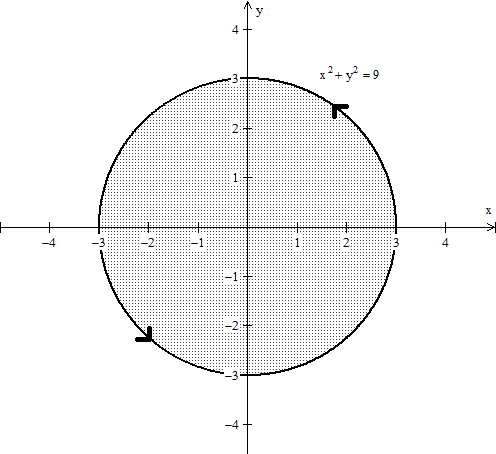• Using polar coordinates allows us to faciliate the integral as r ∈ [0,3] and θ ∈ [0,2π]. Also 8x − 1 = 8rcosθ− 1.
• Hence dydx = ∫003 ( 8rcosθ− 1 ) rdrdθ = ∫003 ( 8r2cosθ− r ) drdθ.
Integrating yields ∫0 ( [8/3]r3cosθ− [(r2)/2] ) |03 dθ = ∫0 ( 72cosθ− [9/2] ) dθ = ( 72sinθ− [9/2]θ ) |0 = − 9π. So ∫C F = − 9π.
Use Green's Theorem to evaluate the integral of the vector field F(x,y) = ( − x2y2,3xy) over the curve C = C1 + C2 + C3 described by: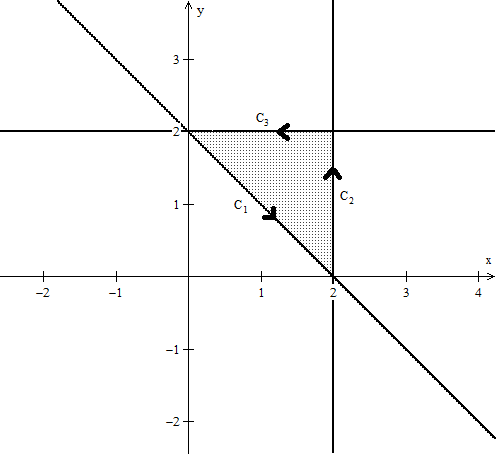• Green's Theorem allows us to find ∫C F by integrating dydx for the region R to the left of the curve C on the vector field F(x,y) = (f1(x,y),f2(x,y)).
• Since f1(x,y) = − x2y2, then [(df1)/dy] = − 2x2y. Also f2(x,y) = 3xy so [(df2)/dx] = 3y. Hence [(df2)/dx] − [(df1)/dy] = 3y − 2x2y.
• To determine our intervals of integration we note that our region R is between the lines y = − x + 2 and y = 2.
• Hence our intervals of integration are y ∈ [ − x + 2,2] and x ∈ [0,2].
• Hence dydx = ∫02 − x + 22 ( 3y − 2x2y ) dydx.
• Integrating yields ∫02 ( [3/2]y2 − x2y2 ) | − x + 22 dx = ∫02 ( [ [3/2](2)2 − x2(2)2 ] − [ [3/2]( − x + 2)2 − x2( − x + 2)2 ] ) dx = ∫02 ( x4 − 4x3 − [3/2]x2 + 6x ) dx
Continuing we have ∫02 ( x4 − 4x3 − [3/2]x2 + 6x ) dx = ( [1/5]x5 − x4 − [1/2]x3 + 3x2 ) |02 = − [8/5]. So ∫C F = − [8/5].
Use Green's Theorem to approximate the integral of the vector field F(x,y) = (5,x + y) over the curve C = C1 + C2 + C3 described by: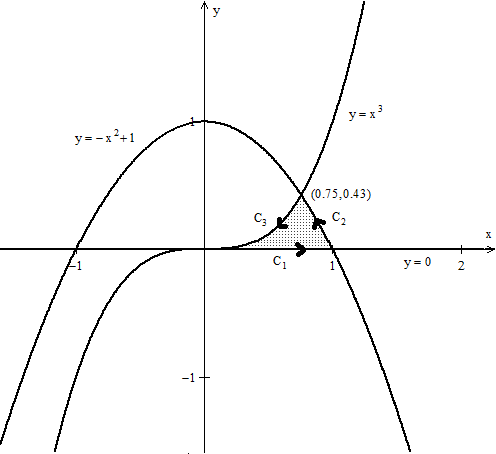• Green's Theorem allows us to find ∫C F by integrating dydx for the region R to the left of the curve C on the vector field F(x,y) = (f1(x,y),f2(x,y)).
• Since f1(x,y) = 5, then [(df1)/dy] = 0. Also f2(x,y) = x + y so [(df2)/dx] = 1. Hence [(df2)/dx] − [(df1)/dy] = 1 − 0 = 1.
• To determine our intervals of integration we note that our region R is between the curves 3√{y} = x and √{1 − y} = x. Hint: solve for x in our given equations.
• Hence our intervals of integration are x ∈ [ 3√{y},√{1 − y} ] and y ∈ [0,0.43].
• Hence dydx = ∫00.433√{y}√{1 − y} 1 dxdy.
Integrating yields ∫00.43 ( x ) |3√{y}√{1 − y} dy = ∫00.43 ( √{1 − y} − 3√{y} ) dy = ( − [2/3]( 1 − y )3 \mathord/ \protect phantom 3 22 − [3/4]y4 \mathord/ \protect phantom 4 33 ) |00.43 >> − 0.53. So ∫C F = − 0.53.
Use Green's Theorem to set up the integral of the vector field F(x,y) = ( − x − y,5) over the curve C = C1 + C2 described by: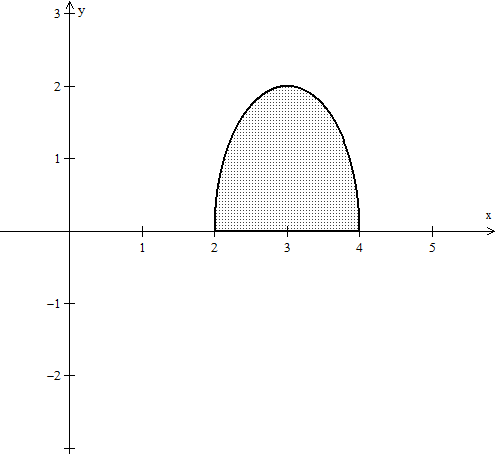• Green's Theorem allows us to find ∫C F by integrating dydx for the region R to the left of the curve C on the vector field F(x,y) = (f1(x,y),f2(x,y)).
• Since f1(x,y) = − x − y, then [(df1)/dy] = − 1. Also f2(x,y) = 5 so [(df2)/dx] = 0. Hence [(df2)/dx] − [(df1)/dy] = 0 − ( − 1) = 1.
• To determine our intervals of integration we note that our region R is between the line y = 0 and y = 2√{1 − (x − 3)2} . Hint: solve for y in our given equation.
• Hence our intervals of integration are y ∈ [ 0,2√{1 − (x − 3)2} ] and x ∈ [2,4].
Hence ∫C F = dydx = ∫2402√{1 − (x − 3)2} 1 dydx.
i) Find the intervals of integration of ∫C F = dA for C = C1 + C2 + C3 + C4 described below using rectangular coordinates and dA = dydx.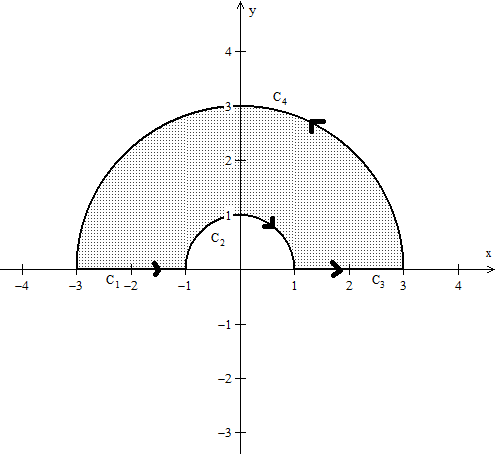• In order to apply Green's theorem with rectangular coordinates, we must split the region R into three components R1, R2 and R3 as shown here
•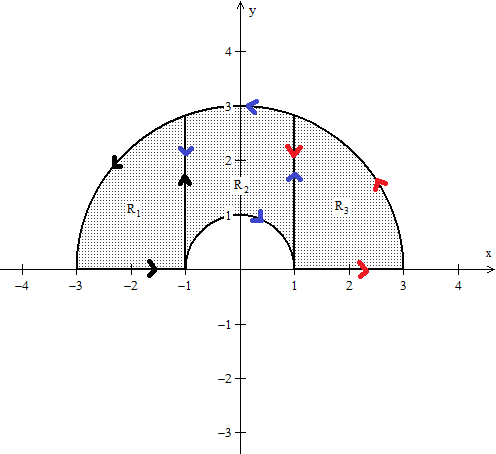• Note that each component has it's own individual path (represented by the black, blue and red arrows) and thus ∫C F = dA + dA + dA.
• Since dA = dydx we look at each region idependently. For R1, the region lies between the line y = 0 and the top semicircle y = √{9 − x2} and x takes the values from − 3 to − 1.
• For R2, the region lies between the top seimicircle y = √{1 − x2} and the top semicircle y = √{9 − x2} and x takes the values from − 1 to 1.
• For R3, the region lies between the line y = 0 and the top semicircle y = √{9 − x2} and x takes the values from 1 to 3.
Thus our intervals of integration yield ∫C F = ∫ − 3 − 10√{9 − x2} ( [(df2)/dx] − [(df1)/dy] ) dydx + ∫ − 11√{1 − x2}√{9 − x2} ( [(df2)/dx] − [(df1)/dy] ) dydx + ∫130√{9 − x2} ( [(df2)/dx] − [(df1)/dy] ) dydx .
ii) Find the intervals of integration of ∫C F = dA for C = C1 + C2 + C3 + C4 described below using polar coordinates and dA = rdrdθ .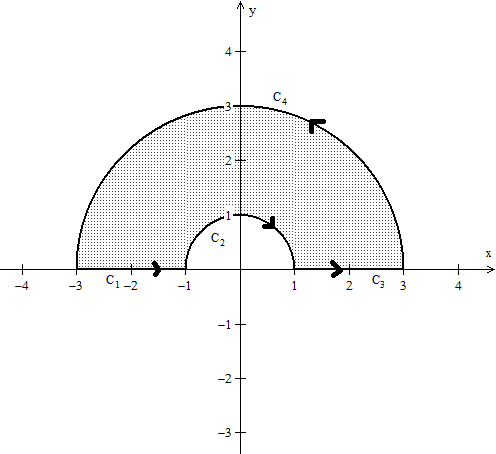• We can apply Green's Theorem with polar coordinates by subtracting the region generated by the circle of radius 3 with the region generated by the circle of radius 1, that is ∫C F = ∫C4 F + ∫C2 F .
• So we have ∫C F = ∫C4 F − ∫C2 F = dA. Note that θ ∈ [0,π] for R and that r ∈ [1,3].
Thus our intervals of integration yield ∫C F = ∫0π13 ( [(df2)/dx] − [(df1)/dy] ) rdrdθ .
Evalute ∫C F for the vector field F(x,y) = (x2 − y,y2 + x) over the curve C described below. Hint: ∫cos2u du = [1/2]u + [1/4]sin(2u) + C: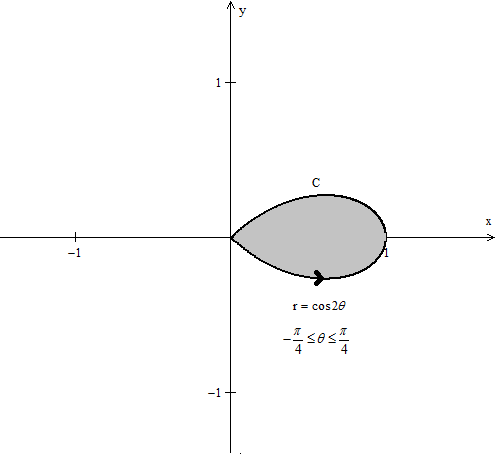• Using Green's Theorem we have ∫C F = dA.
• Now f1(x,y) = x2 − y and so [(df1)/dy] = − 1. Also f2(x,y) = y2 + x so [(df2)/dx] = 1. Hence [(df2)/dx] − [(df1)/dy] = 1 − ( − 1) = 2.
• Using polar coordinates faciliates the integral as r ∈ [0,cos2θ] and θ ∈ [ − [(π)/4],[(π)/4] ].
• Hence dA = ∫ − π \mathord/ phantom − π 4 4π\mathord/ phantom π4 40cos2θ 2 rdrdθ.
Integrating yields ∫ − π \mathord/ \protect phantom − π 4 4π\mathord/ \protect phantom π4 4 ( r2 ) |0cos2θ dθ = ∫0 cos22θ dθ = [1/2]( [1/2](2θ) + [1/4]sin(2(2θ)) ) | − π \mathord/ \protect phantom − π 4 4π\mathord/ \protect phantom π4 4 = [1/2]( θ+ [1/4]sin(4θ) ) | − π \mathord/ \protect phantom − π 4 4π\mathord/ \protect phantom π4 4 = [(π)/4]. So ∫C F = [(π)/4].
Evalute ∫C F for the vector field F(x,y) = (1 − cos(x),sin(y)) over the curve C described by: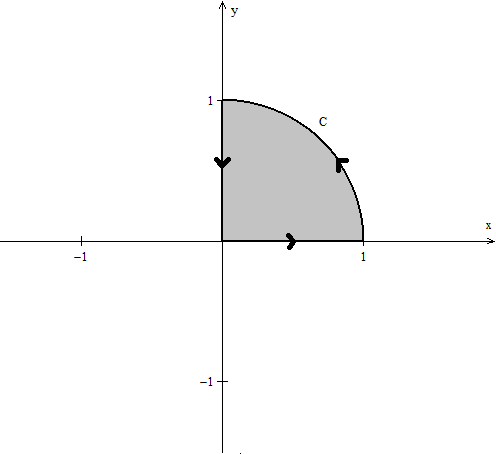• Using Green's Theorem we have ∫C F = dA.
• Now f1(x,y) = 1 − cos(x) and so [(df1)/dy] = 0. Also f2(x,y) = sin(y) so [(df2)/dx] = 0. Hence [(df2)/dx] − [(df1)/dy] = 0.
• It follows that no matter what our region of integration is, the integral will always yield zero.
Hence dA = 0.
Evalute ∫C F for the vector field F(x,y) = (ey,xey) over the curve C described by: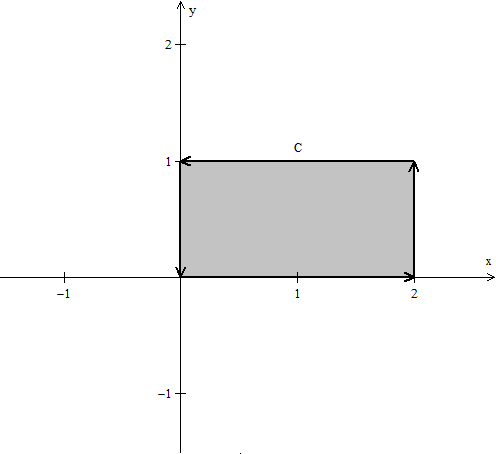• Using Green's Theorem we have ∫C F = dA.
• Now f1(x,y) = ey and so [(df1)/dy] = 0. Also f2(x,y) = xey so [(df2)/dx] = ey. Hence [(df2)/dx] − [(df1)/dy] = ey − (0) = ey.
• Using rectangular coordinates faciliates the integral as y ∈ [0,1] and x ∈ [ 0,2 ].
• Hence dA = ∫0201 ey dydx.
Integrating yields ∫0201 ey dydx = ∫02 ( e − 1 ) dx = ( xe − x ) |02 = 2e − 2. So ∫C F = 2(e − 1).
Set up ∫C F for the vector field F(x,y) = (x3 − y3,x2 + y2) over the curve C described below in polar coordinates.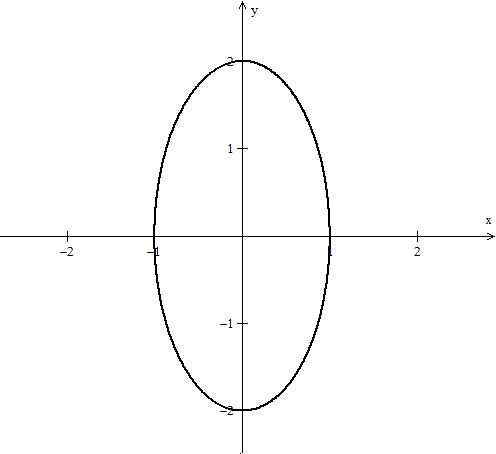• Using Green's Theorem we have ∫C F = dA.
• Now f1(x,y) = x3 − y3 and so [(df1)/dy] = − 3y2. Also f2(x,y) = x2 + y2 so [(df2)/dx] = 2x. Hence [(df2)/dx] − [(df1)/dy] = 2x − ( − 3y2) = 2x + 3y2.
• Using polar coordinates gives θ ∈ [0,2π] and r ∈ [ 0,[2/(√{4cos2θ+ sin2θ} )] ]. Note, convert x2 + [(y2)/4] = 1 into polar terms and solve for r.
• Also 2x + 3y2 = 2(rcosθ) + 3(rsinθ)2 = 2rcosθ+ 3r2sin2θ.
• Hence dA = ∫00[2/(√{4cos2θ+ sin2θ} )] ( 2rcosθ+ 3r2sin2θ ) rdrdθ.
Our integration is thus ∫00[2/(√{4cos2θ+ sin2θ} )] ( 2r2cosθ+ 3r3sin2θ ) drdθ .
Evalute ∫C F for the vector field F(x,y) = (x − y,x + y) over the curve C described by: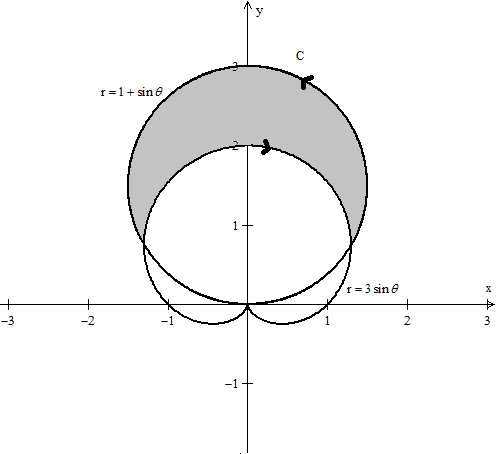Hint: ∫sin2u du = [1/2]u − [1/4]sin(2u) + C
• Using Green's Theorem we have ∫C F = dA.
• Now f1(x,y) = x − y and so [(df1)/dy] = − 1. Also f2(x,y) = x + y so [(df2)/dx] = 1. Hence [(df2)/dx] − [(df1)/dy] = 1 − ( − 1) = 2.
• Using polar coordinates faciliates the integral as θ ∈ [ [(π)/6],[(5π)/6] ] and r ∈ [ 3sinθ,1 + sinθ ]. Note that to find the intervals of integration for θ, we eθuate 3sinθ with 1 + sinθ and solve for θ .
• Hence dA = ∫π\mathord/ phantom π6 65π \mathord/ phantom 5π 6 63sinθ1 + sinθ 2 r drdθ .
• Integrating yields ∫π\mathord/ phantom π6 65π \mathord/ phantom 5π 6 63sinθ1 + sinθ 2 r drdθ = ∫π\mathord/ phantom π6 65π \mathord/ phantom 5π 6 6 r2 | 3sinθ1 + sinθdθ = ∫π\mathord/ phantom π6 65π \mathord/ phantom 5π 6 6 ( 1 − 2sinθ− 8sin2θ ) dθ = ( θ+ 2cosθ− 8[ [1/2]θ− [1/4]sin(2θ) ] ) |π\mathord/ phantom π6 65π \mathord/ phantom 5π 6 6
Hence ∫C F = ( − 3θ+ 2cosθ+ 2sin(2θ) ) |π\mathord/ protect phantom π6 65π \mathord/ \protect phantom 5π 6 6 = − 2π− 4√3 .

*These practice questions are only helpful when you work on them offline on a piece of paper and then use the solution steps function to check your answer.

### Green's Theorem

Lecture Slides are screen-captured images of important points in the lecture. Students can download and print out these lecture slide images to do practice problems as well as take notes while watching the lecture.

• Intro 0:00
• Green's Theorem 0:38
• Introduction to Green's Theorem and Notations
• Green's Theorem
• Example 1: Find the Integral of the Vector Field around the Ellipse
• Verifying Green's Theorem with Example 1
• A More General Version of Green's Theorem
• Example 2
• Example 3
• Example 4

### Transcription: Green's Theorem

Hello, and welcome back to educator.com and multivariable calculus.0000

So, today's lesson we are going to be talking about Green's Theorem. Absolutely fantastic theorem. Very, very important.0004

Green's theorem is the fundamental theorem of calculus in 2 dimensions.0011

Single variable calculus, you did the fundamental theorem of calculus. In 2 dimensions it is called Green's theorem. In 3 dimensions it is called Stoke's theorem.0017

As it turns out, the fundamental theorem of calculus it actually true in any number of dimensions, and the name of that is called the generalized Stoke's theorem.0025

So, let us just jump in, and work some examples. Okay. So, let us start off with by defining what it is that we are going to be doing here.0035

So, let f(x,y) = f1(x,y) and f2(x,y), so this is just the standard vector field that we have been dealing with all along.0045

This is the first coordinate function, and this is the second coordinate function of your basic vector field.0063

Let it be a vector field -- okay, oops, go ahead and put this here -- and let c(t) be a curve.0069

Again, nothing new, we have been dealing with this all along.0090

Now, we said that the line integral, so the integral of f along c, well that is equal to the integral from a to b, a to b is the interval over which the curve is defined, of f(c(t)) · c'(t) dt.0095

Your standard line integral that we have been doing all along. Well, we also introduced another notation for this, so I am going to go ahead and use this particular notation and the reason I am going to use it... I am sort of going to be going back and forth when I discuss line integrals with respect to Green's theorem.0119

The reason I am going to be doing that is most books, they use the notation that I am about to show you right now. We have seen it before, but we have not really used it all that much, but just to remind you, so we also introduced the notation instead of this, the integral of c, the integral of f/c... we also used the notation f dx + g dy.0136

In this particular case, since we are using f1 and f2, it is going to be f1 dx + f2 dy. That is it. It is just another notation that is used for representing the line integral.0175

The actual evaluation of the integral is this one. This is the most important part.0192

Okay. So, now I will go ahead and I will state Green's theorem.0198

Let f1 and f2 be continuous -- hmm, let me write continuously a little bit better than this -- be continuously differentiable functions over a region R and let c be the boundary of that region.0205

In other words, c is a closed curve. So, in other words, c is a closed path, a closed curve -- I will write closed path here... like that.0260

If we traverse the boundary, if we traverse c in the counterclockwise direction... this is very, very important... in the counterclockwise direction so that R, the region, is always to the left of the curve... is always left of c.0276

Then, and here is the big one -- move to the next page to actually write this one out -- the integral f1 dx + f2 dy = the double integral over the actual region of... I am going to write this a little bit better... df2 dx - df1 dy dy dx.0316

So, let me go ahead and draw the region and I am just going to talk about it a little bit here.0362

Let us just make this region like that. This is our region R, and this is our curve c, which happens to be the boundary of that region.0367

We are traversing it in this direction. We are moving this way, and as we move this way, the region is always going to be to the left of the curve so there is orientation. It does matter actually, how this is done.0376

When this happens. When you have a given region in 2-space, and when you have these functions f1 and f2 defined over this region, and if you actually have a closed path that contains this particular region, this Green's theorem, here is what it is saying.0389

It is saying that if you take the integral, the line integral of this function along this path which we have done already, that is actually equal to the double integral of this thing that if you take the derivative of... if you take the partial derivative of the second function with respect to x, subtract the partial derivative with respect to y of the first function and if you integrate this thing that you get, okay? over the area that is actually enclosed by this curve, those 2 numbers are the same.0406

This is profoundly important. Actually it is kind of extraordinary. It is expressing a relationship between the boundary of a region and the area that that boundary contains.0439

From a purely theoretical, global standpoint, that is what this is saying.0450

It says if I -- let us say I have a line integral, but for some odd reason this line integral is really, really difficult to evaluate, but I need that line integral.0455

Well, it says it might be easier if I just actually do a double integral over the area contained by that path. That is what Green's theorem says.0465

Again, the integral of f1 dx + f2 dy, which is the integral of the particular function, a vector field, the line integral is equal to the double integral of this integrand, df2 dx - df1 dy, dy dx.0475

Instead of doing a line integral, I can do a double integral... and vice-versa. Let us say I need a double integral, if over a particular region but for some odd reason I... it is difficult or I do not want to do it... if I can some how find a boundary then I can go ahead and do the line integral instead. Green's theorem. Profoundly important.0492

Okay. Let us just go ahead and do an example here. I think that is the best way to approach this.0512

Now, we are going to have a lot to say about Green's theorem and the 3-dimensional version which is called Stoke's theorem.0518

We are actually going to be talking about it a fair amount because this is profoundly important in engineering, in physics, in mathematics... I mean this is the fundamental theorem of calculus.0526

I mean, it is huge... but we want to develop some facility with it, and again, by gaining some facility with it we will get more comfortable with it and then once we are reasonably comfortable dealing with it technically, we can start to think about it more theoretically. That is what we want.0535

Okay. So, Example 1. Find the integral of the vector field -- I am just going to write "vf" for vector field -- of the vector field f which is equal to x2y, xy3 around the ellipse x2 + 4y2 = 16.0552

So, we have this ellipse -- it is going to look something like this -- or actually, no, I am sorry, let us go ahead and put this into standard form just so you are accustomed to seeing it.0593

This is going to be x2/42 + y2/22 = 1, that is the standard form, and yes, I apologize.0611

It looks like that, it is going to be an ellipse that is centered at the origin. Its major focal radius is going to be 4 this way, minor focal radius is going to be 2 that way.0622

We want to find the integral of this vector field over around the ellipse, so this is a line integral we are asking for, not the region. We are going to do the region.0634

We are going to use Green's theorem, and integrate over the region that is contained by this ellipse.0644

What we want is the line integral of this vector field around the ellipse. Okay. Let us go ahead and do this.0648

Let us see. So, let us go ahead and write Green's theorem again, because it is always a great idea to write down the actual theorem itself over and over again, until you become accustomed to it.0657

So I am going to use, the integral of f/c is going to be the double integral over a -- I will call this region that the ellipse contains a -- of d1 so this is f1, this is f2, d1(f2) - d2(f1). That is another way of writing this.0670

df2 dx - df1 dy in capital D notation, this is d1f2 - d2f1, right? Take the derivative with respect to the first variable of the second function, the derivative with respect to the second variable of the first function.0705

Okay. dy, dx, this is Green's theorem. We are not going to evaluate the line integral, we are going to go ahead and do it as an area integral.0727

Let us see what is going on here. Well, let us go ahead and first of all, see how we are going to integrate. We are going to integrate over this region.0738

Well, we need to know upper and lower limits here. Well, we know that this is going to be -4, and we know that this is 4. I am going to integrate in the x direction from -4 to 4. That is going to be my dx, the upper lower limits of integration with respect to x.0746

As far as y is concerned, I need to find the difference from here. So, this is a function and this upper part is a function.0763

I am going to take the... this is going to be my lower limit, that is going to be my upper limit, so I need to express... turn this equation for the ellipse into an explicit relation between y and x.0776

Let me go ahead and do that first. So, I have got x2 + 4y2 = 16.0790

I have got y2 = 16 - x2, all divided by 4, and then when I take the square root of that, I have got + or - the square root of 16 - x2/2.0800

My upper integration is going to be sqrt(16) - x2/2, I am sorry, my lower is going to be the negative of that.0823

Let me just write what this integral actually looks like.0835

Okay. Before we do that we need to find out of course what d1(f2) - d2(f1) is.0840

Let us remind ourselves, we have x... what was our... I think we had x2y, and we had xy3, was that our...? yes, that is correct.0850

So, the derivative of f2 with respect to x is going to be y3 - the derivative with respect to y of f1, which is x2.0865

The integral of f/c is equal to -4 to 4 -sqrt(16) - x2/2 to + sqrt(16) - x2/2.0879

d1f2 is d2f1, we get y3 - x2 dy dx.0906

So, in this particular case we are using Green's theorem. They wanted the integral of our vector field around the ellipse. Well, what we decided to do is to use Green's theorem and calculate it as a double integral.0914

Well, when we go ahead and actually put this integral into our mathematical software, we get - 32pi. That is it.0927

Now what I am going to do for this particular problem, I am actually going to go back and evaluate this line integral directly, just to verify that they are actually the same. That we are going to end up with a -32pi. Let us go ahead and do that.0937

So, let us verify Green's theorem in this case by calculating the line integral directly.0949

I tell you, I love math software precisely for this reason. It really lets you concentrate just on the math, and takes care of all of the technical details for you.0974

I mean, you remember when you were in calculus... so much of the time that was spent in calculus was just algebra, and converting, converting, converting... I mean you could have 1, 2, 3, pages of just... you know... calculations and conversions.0984

It was not really calculus itself, I mean calculus was the integral. It is this that is important, the rest, we should not have to spend all of our time. Unfortunately now we have software, we do not have to spend all of our time doing it.0998

So, let us remind ourselves what it is that we have. We have our vector field, which is x2y and xy3, this is cubed, and we have our c(t), so in this particular case we need the parameterization of the ellipse.1011

Well, you remember the parameterization of the ellipse... 4cos(t), 2sin(t), or acos(t), bsin(t) where a and b are the focal radii, the major and minor focal radii of the ellipse.1029

We have to have a parameterization because that is how we solve a line integral, and of course t runs from 0 to 2pi, or another notation for that is t is from the integral from 0 to 2pi.1045

Now, let us go ahead and calculate f(c(t)). I am going to put these values into here and I am going to get 32cos2(t), sin(t), and I am going to get 32cos(t), sin3(t).1064

Again, this is f(c(t)), this is f, this is c(t), we have done this 1000 times before.1086

Now, let us do c'(t). So c'(t), which I prefer to write as dc dt, just a personal choice, we have -4sin(t), you are just taking the derivative of the curve and 2cos(t).1092

Then of course when I take the dot product of this, and this, so... when we have f(c) dotted with c', that is going to equal -128cos2(t), sin2(t) + 64cos2(t), sinc3(t).1108

This is actually going to be our integrand, so let us go ahead and calculate this line integral. I will do it on this page here.1140

So, the integral of f around c is equal to the integral from 0 to 2pi of this thing that we just had... -128cos2(t), sin2(t) + 64cos2(t),sin3(t), very, very complicated but again, we just put it into our math software and we get -32pi.1150

This confirms it. The line integral around a closed path was equal to the double integral of this thing df2 dx - df1 dy, we will talk more about what this thing is in the next lesson, over the area enclosed by that ellipse.1181

Fantastic. Okay. So, now a more general version of Green's theorem.1202

A more general version of Green's theorem allows the boundary of a given region "a" to be composed of a finite number of curves.1223

It does not have to be a single curve, like a circle, or an ellipse, or some continuous shape.1249

It could be composed of a series of finite number of curves, and we will do a couple of examples in just a minute... composed of a finite number of curves.1261

c1, c2, all the way to cn, not just a single differentiable curve.1273

So, I will do like a quick, so we are going to have something that looks like this. That is 1, that is 2, so that, that is another... that is another... so we might have this is c1, this is c2, this is c3, c4, c5.1297

This is a perfectly good region and the boundary can be parameterized by any number of... you know... any finite number of curves.1315

Here is the best part. These curves do not actually have to be connected end to end. That is the best part. So, that is very, very important to note and I will put it down here.1322

Note these curves do not necessarily need to be connected end to end, like this particular region.1333

This is this region in a, right there. Do not need to be connected end to end, and end to end means piecewise continuous.1353

So, these are all continuous, they are not just continuous, they are piecewise continuous. It does not have to be.1368

Our next example is going to be an example of that, so let us go ahead and do example 2 here. Example 2.1376

Let us go ahead and draw out this particular region then. Something like this... if I have that circle, let us say I have a circle that is a little bit bigger.1391

Well, if the region that I am interested in, region a, happens to be the area that is actually in between those circles, well, this is c1 and this over here is going to be c2.1409

The boundary of this region is 2 curves. In this case I have 2 curves, but the curves are not connected end ot end.1424

This is perfectly valid. This is a perfectly good region, and Green's theorem actually applies, but remember what we said about Green's theorem.1432

It says that as you traverse the boundary, the region that you are interested in has to be to your left.1440

In this particular case, it says that if you traverse this particular curve, this in this direction, well yes this region is definitely to our left.1445

Here if we go in the counter clockwise direction on this region... I am sorry, along this curve... now the region is to our right.1453

All we have to do is traverse it in the reverse direction. We need to go clockwise for the inner, and when we go clockwise, when we go in this direction, now the region is actually going to be on our left and Green's theorem applies.1462

That is it. That is all that is happening here. So, let us see. In this particular case, c, the boundary is equal to c1 + c2, except c2 traversed in the opposite direction.1477

That is what this little negative sign means. Remember? We talked about that a little bit. The only difference is if you traverse something in the opposite direction, all you are doing is you are changing the sign of the integral.1491

So it does not really matter how you traverse it, all you have to do is remember the direction in which we are traversing it, and then change the sign of the integral.1502

In this case, we have the following. We have the integral of f along c is equal to the integral of f/c1 + the integral of f/c2 -, which is equal to the integral of f along c1, because that is counterclockwise, and we just change the sign... - the integral of f along c2.1510

That is equal to well, the double integral over a of d1f2 - d2f1 dy dx. Green's theorem applies, it still applies.1543

Take the particular vector field, I form this little thing, d1f2 - d2f1, and I integrate over that particular region, in this particular case we are talking about two circles, it is probably easiest to do it in our polar form.1563

But, again, we have math software, you can do it in any form you like. The important point is that the boundary curve of the particular region, they do not have to be connected. That is what is important.1578

Alright, so let us do an example. Example 3. We will let f equal to, again we will use our same vector field, we will use x2y and xy3, and a be the region of points xy such that x2 + y2 is greater than or equal to 1, and less than or equal to 9.1591

So, what this is saying is that we are going to integrate this vector field over the region in between the circles, the unit circle of radius 1 and the circle of radius 3. That is it.1640

Let us go ahead and draw our region. So, this right here, this stipulation... unit circle, radius 1, 9 is going to be a circle of radius 3, so here, radius = 3, here radius = 1.1652

We want to integrate the region in between there. That is it. Okay. So, let us go ahead and do this, and we said probably this was going to be easiest in polar coordinate form.1673

Simply because the equation for circles is really, really easy in polar coordinates. In this particular case, R = 1 and R = 3, that is it, and θ is 0 to 2pi, right?1684

We are sweeping out, so R goes from 1 to 3, so we are sweeping out this line out this way, all the way around, 0 to 2pi, θ is in 0 to 2pi, that is it.1701

Okay. Let us go ahead and work this problem out. The first thing we want to do is we want to find of course the d1f2 - d2f1, which is the same as df2 dx - df1 dy, so just different notations for the same thing.1715

This was the previous vector field, so we got y3 - x2.1737

Well, we want to do this in polar form so we are going to have to actually convert y3 - x2 because we are taking f(t), so let us write everything out here.1745

So, the integral of f/c is going to equal the double integral over a of y3 - x2 dy dx. That is Green's theorem.1759

Well, so this is f this is that, this is the region, so we are going to express this in polar form. We need to express this in polar form.1776

In other words we need to find this f(t), where t is x = Rcos(θ), y = Rsin(θ).1785

Therefore our f(t), this thing, when we convert it to polar form -- oops, f of capital T -- T is going to equal, well, y3 is R3sin3(θ) - x2, which is R2cos2(θ).1800

So, we have... the integral... well, we are going -- now, this is going to be the double integral over a expressed in polar form of f(t) R dR dθ.1826

So θ is going to run from 0 to 2pi, right? we are running around, and our R goes from 1 to 3.1844

Our f(t) is R3sin3(θ) - R2cos2(θ), and then we have our area element... R dR dθ. That is our integral.1856

When we do this, we end up with -20pi.1874

That is it. We had a region, consisted of the boundary of that region, consisted of two curves, this way, and these 2 curves right here. They are not connected... Green's theorem is still valid, we can still go ahead and do the double integral... very, very nice.1882

I mean, yeah, it is fine, these are circles, so we certainly could have done the line integral ourselves. We have the line integral over this one, and then we take the line integral here, we go in the reverse direction, we change the sign of it and then we can add it and get the same number.1903

I would actually recommend that you do that to confirm that you still end up with the number -20pi, but that is the beauty of Green's theorem, I do not need to do the line integral, I can solve it as a double integral.1914

Okay. let us close off with one more example just to solidify everything in our minds.1926

So, we have example 4. Now, this time we will let f equal to 2xy and cos(xy).1934

Well, our task is to find the integral of f/c where c = the 2 curves c1 and c2, where c1 is equal to sin(x), and c2 is equal to sin(2x).1950

We would like x to range from 0 to pi, so let us go ahead and draw out what this region is.1977

So, we have the sine function, y = sin(x), this is 1 and -1, right? I am going to draw it a little bigger here, so... 2 is here, let us see... 2sin(x), actually I think I have 2x, 2sin(x)... umm, yes, I am sorry, this is not sin(2x), this is 2sin(x). There we go.1990

So, sin(2x) would actually end up giving me a different period. We are going to have the same period, so basically, I have sin(2x), and I do this one and it is something like this.2030

We want to go from 0 to pi, so from 0 to pi, so this is the region right here. The region -- so, this is one curve, this is the other curve, so basically what they are asking me to do is we want to find the line integral of this vector field around this path.2042

I have not drawn it very well but that is it. So, instead of doing the line integral, we are going to use Green's theorem and we are going to integrate this vector field over the area enclosed by that path. That is what we are doing.2075

Okay, so let us go ahead and just work it out. Well, so this is f1, and this is f2, we want to find -- let me see, let us go ahead and do df2 dx, that is going to be... so if I take this, the derivative of this with respect to x I am going to get -y × sin(xy).2090

If I take df1 dy, I am going to end up with 2x, therefore my integrand... so this minus that... so my df2 dx - df1 dy, which is my integrand under my double integral, is going to equal minus y × the sin(xy) - 2x.2121

Now we are integrating x, so our line integral of f/c is equal to... well we are going from 0 to pi, so as far as x is concerned, it is going to be 0 to pi, and as far as y is concerned, the region is between the 2 graphs, sin(x) and 2sin(x).2156

We have -y × the sin(xy) - 2x, that is our integrand and then we have dy dx, and I am actually going to let you work this out. Hopefully you have some mathematical software and see what that particular number is.2193

Again, doing it is not as important as actually being able to take a particular problem, find a region, find the boundary of that region, decide what the upper and lower limits of integration are, if you are in fact going to use the Green's theorem to solve a double integral instead of a line integral.2214

We can certainly use a line integral here, it is not a problem. We can parameterize both of these curves and do each one separately and add them, but we might as well avail ourselves of Green's theorem since it is there for us.2233

We take df2 dx - df1 dy, that is our integrand, we have our limits of integration 0 to pi, sin(x) to 2sin(x)... and that is about it. The rest is just a question of plugging it into your software and getting some number. That is it.2243

That is Green's theorem. Now we definitely are going to have more to say about Green's theorem, no doubt about it.2261

In fact, next lesson, we are going to go a little bit deeper and talk about 2 different version of Green's theorem -- something called the circulation curl version, and something called the flux divergence version.2266

Until then, thank you for joining us here at educator.com, we will see you next time. Bye-bye.2278

OR

### Start Learning Now

Our free lessons will get you started (Adobe Flash® required).